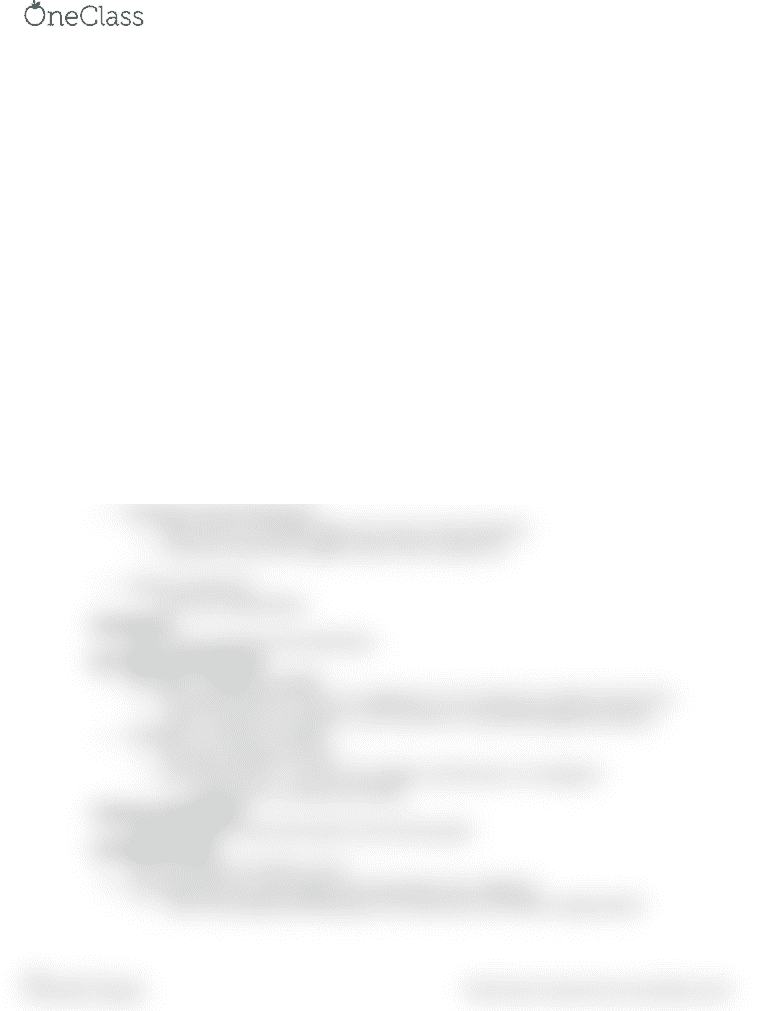# PSYCH 100A Lecture Notes - Lecture 3: Central Tendency, Standard Deviation

69 views2 pagesDistributions
Can be a graph/table/mathematical function describing the frequency of each score
Frequency describes how often something occurs
The normal distribution is a theoretical function that describes many physical and
psychological traits
o Apex of the curve is the highest occurrence at the middle
Center of distribution is important
Typical score
o Lower tail/upper tail is where the curve levels off
Region where uncommon scores occur
o Bell-curve
Theoretical vs. Empirical Distributions
Center: represents typical response, lower spread (high peak)
Spread - (or variability) like standard deviation, sample of scores where people are more
similar to one another. Low peak
o High variability - low peak
o Low variability - high peak, scrunched up
Shape - depends on where the peak is
o In what area do most scores fall?
Positively skewed distribution
o Most scores in the low range, high scores are less frequent
o Tail point towards the positive side of the number line
Negatively skewed distribution
o Most scores in the high range, low scores are less frequent
o Tail points toward the negative side of the number line
Uniform distribution
o All scores equally likely
Distributions
How can we summarize the distribution
Bar Graphs and Histograms
Bar graphs: categorical variable
o Put spaces between the bars to categorize it, not continuous number line but bins
o Order of the bars are arbitrary. Can't say that it is positively/negatively skewed.
Histograms: continuous variable
o No spaces between the bars
o Kernel Density Plot - connects the heights of all the bars in a histogram
Represents a continuous function
Frequency Distributions
A tabular display of the information from the bar graph
Cumulative Percent
The percentage at or below a score
Accumulate the two percentages from the bottom two categories
o "15% of the people participating in the study were at or below inappropriate"
find more resources at oneclass.com
find more resources at oneclass.com
Unlock document

This preview shows half of the first page of the document.
Unlock all 2 pages and 3 million more documents.